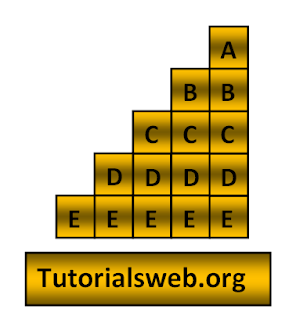## Write "Java"  Program to print below Alphabet Pattern19 (or) Character Pattern19:To understand this example, you should have knowledge of the following topics:

·   Java programming operators.

Java Character pattern 19 program using For loop:
`import java.util.Scanner;`
`class alphabetPattern19`
`{`
`public static void main(String[] ags)`
`{`
`    int i, j,k,n;`
`   System.out.println("Enter the number of lines");`
`   Scanner s = new Scanner(System.in);`
`   k = s.nextInt();`
`    for(i = 1;i <= k;i++)`
`    {  `
`        for(j = 1;j <= k-i;j++)`
`           {`
`            System.out.print(" ");`
`            }`
`           for( n = 1; n <= i; n++)`
`           {`
`            System.out.print((char)(i+64));`
`            }`
`        System.out.println();`
`    }`
`  }`
`}`
Output:
```
Enter the number of lines
5
A
BB
CCC
DDDD
EEEEE
```
Explanation:
• First four integers "i", "j", "k","n" are declared of type int.
• Then in the First for loop “i” value is initialized with a value of "1".
• Now “i” value is checked with the condition "i <= k" (where "k" value is given through Keyboard). If the condition is True loops enter into second for loop.
• Now the loop enters into second for loop and checks the condition "j <= k-i". Where "j = 1". and the condition is True prints the statement.
• Now the loop enters into third for loop and checks the condition "j <= i". Where "n = 1". and the condition is True prints the statement.
• Now prints the " Alphabet". Print up to third for loop condition becomes False.
• When the value of "k"  becomes less than "i" value the program terminates.

## Search This Blog

### C Variables and Types of Variables in C

C variable is a data name that may be used to store a data value. The value of the C variable may get change in the program and it can be r...